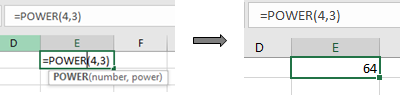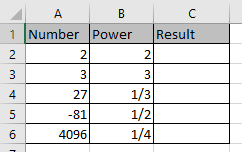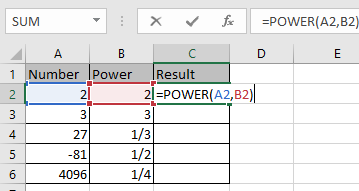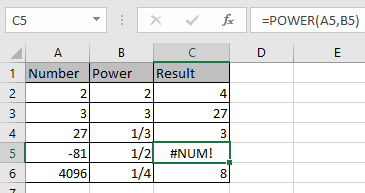# How to use the Excel POWER function

In this article, we will learn how to use the POWER function in Excel.

In mathematics power operator, gets the power of a number.

33= 3 * 3 *3 = 27
3 raised to the power 3, says 3 multiplied by its own number three-time is equal to 27.

271/3= 33*(1/3)= 3
27 raised to the power (?), says which number gets multiplied 3 times to get 27, 3 is the answer. This is called finding the root of a number.

POWER function calculates the power of a number.

Syntax:

=POWER ( number, power)

For example: IF you wish to get the power of 4 raised to 3
Write the formula in the cell =POWER(4,3)Let’s learn this through an example shown below.
Here we have numbers in one column and power of the numbers in the second column.Use the formula to get the POWER

=POWER(A2,B2)Press Enter and copy the formula in other cells using Ctrl + D shortcut.As you can see the formula returns #NUM error when we calculate the root of a negative number.

Hope you learned how to get the power or root of a number using POWER function in Excel. Explore more articles here on Excel Mathematical functions here. Please feel free to state your query or feedback in the comment section below. We will help you.

Related Articles

How to use the POWER Function in Excel

Find the nth root of a number

How to use the IMCONJUGATE Function in Excel

How to use the IMEXP Function in Excel

How to use the IMSIN Function in Excel

How to use the IMSUM Function in Excel

How to use the IMSUB Function in Excel

How to use the IMARGUMENT Function in Excel

How to use the IMCOS Function in Excel

Popular Articles

50 Excel Shortcut to Increase Your Productivity

Edit a dropdown list

If with conditional formatting

If with wildcards

Vlookup by date

Terms and Conditions of use

The applications/code on this site are distributed as is and without warranties or liability. In no event shall the owner of the copyrights, or the authors of the applications/code be liable for any loss of profit, any problems or any damage resulting from the use or evaluation of the applications/code.